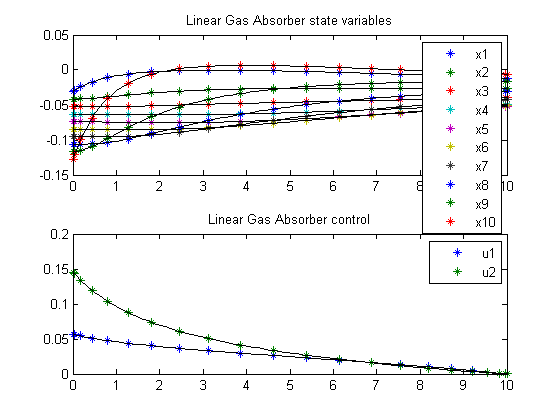58  Linear Gas Absorber

ITERATIVE DYNAMIC PROGRAMMING, REIN LUUS

Section 7.4.4 Gas absorber with a large number of plates

CHAPMAN & HALL/CRC Monographs and Surveys in Pure and Applied Mathematics

58.1  Problem description

A general case of an n-plate gas absorber controlled by inlet feed stream concentrations

Find u over t in [0; 10 ] to minimize

J =
 10 0
x′*x + u′*u dt

subject to:

 dx dt
= A*x + B*u

A = tridiag(0.538998 -1.173113 0.634115)

B = [0.538998 0 ... 0 0; 0 0 ... 0 0.634115]

The initial condition is chosen as:

xi(0) = -0.0307-(i-1)/(n-1)*(0.1273-0.0307), i= 1, 2, ... , n. where n is the number of stages

 0 <= u1 <= inf
 0 <= u2 <= inf

Reference: 

58.2  Problem setup

toms t
n   = 10;
t_f  = 10;

p = tomPhase('p', t, 0, t_f, 20);
setPhase(p);

x = tomState(p, 'x', n, 1);
u = tomControl(p, 'u', 2, 1);

i = (1:n)';
x0i = -0.0307-(0.1273-0.0307)/(n-1)*(i-1);

% Initial guess
% Note: The guess for t_f must appear in the list before expression involving t.
guess = icollocate(x == x0i);

% Box constraints
cbox = {0 <= collocate(u)};

% Initial conditions
cinit = initial(x == x0i);

% Various constants and expressions
A = spdiags([0.538998*ones(n,1) ...
-1.173113*ones(n,1) 0.634115*ones(n,1)],-1:1,n,n);
B = sparse(n,2);
B(1,1)   = 0.538998;
B(end,2) = 0.634115;

% ODEs and path constraints
ceq = collocate(dot(x) == A*x+B*u);

% Objective
objective = integrate(x'*x+u'*u);

58.3  Solve the problem

options = struct;
options.name = 'Linear Gas Absorber';
solution = ezsolve(objective, {cbox, ceq, cinit}, guess, options);

% Extract optimal states and controls from solution
Problem type appears to be: qp
Starting numeric solver
===== * * * =================================================================== * * *
TOMLAB - Tomlab Optimization Inc. Development license  999001. Valid to 2011-02-05
=====================================================================================
Problem:  1: Linear Gas Absorber                f_k       0.328245958214705650
sum(|constr|)      0.000000000000006954
f(x_k) + sum(|constr|)      0.328245958214712590
f(x_0)      0.000000000000000000

Solver: CPLEX.  EXIT=0.  INFORM=1.
CPLEX Barrier QP solver
Optimal solution found

FuncEv   15 GradEv   15 ConstrEv   15 Iter   15
CPU time: 0.109375 sec. Elapsed time: 0.062000 sec.

58.4  Plot result

subplot(2,1,1)
ezplot(x);
legend('x1','x2','x3','x4','x5','x6','x7','x8','x9','x10')
title('Linear Gas Absorber state variables');

subplot(2,1,2)
ezplot(u);
legend('u1', 'u2');
title('Linear Gas Absorber control');